Next: Flux Quantization and the Up: Quantum Dynamics Previous: Charged Particle Motion in

# Gauge Transformations in Electromagnetism

In the Schrödinger picture, the Hamiltonian (3.71) leads to the following time-dependent wave equation: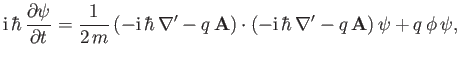(3.89)

where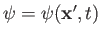,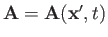, and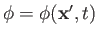. Now, the Heisenberg equation of motion (3.88) only involves the electric and magnetic fields, and is independent of the vector and scalar potentials. On the other hand, the previous wave equation involves the potentials, but not the fields. As is well known, the vector and scalar potentials are not well defined, in that there are many different potentials that generate the same electric and magnetic fields. To be more exact, a transformation of the formand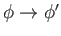, where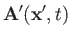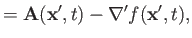(3.90)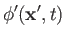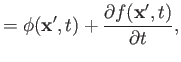(3.91)

and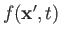is an arbitrary function, leaves the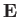and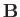fields unaffected. Such a transformation is known as a gauge transformation. It is evident that a gauge transformation would leave the Heisenberg equation of motion (3.88) unchanged, but would modify the time-dependent wave equation (3.89). However, these two equations are supposed to give results that are consistent with one another. Let us investigate how this is possible.

The previous three equations can be combined to give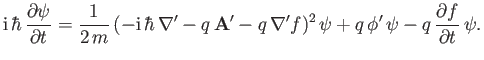(3.92)

Let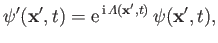(3.93)

where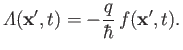(3.94)

It follows that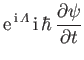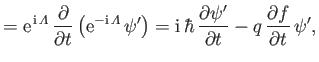(3.95)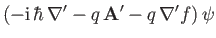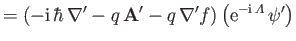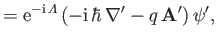(3.96)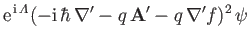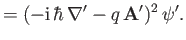(3.97)

Hence, Equation (3.92) becomes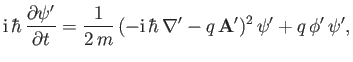(3.98)

which is analogous in form to Equation (3.89). Thus, we deduce that ifandthen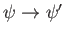. In other words, a gauge transformation introduces a position- and time-dependent phase-shift,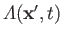, into the wavefunction.

Now, Equation (3.88) is equivalent to Equations (3.76) and (3.87). If we take the expectation values of the latter two equations then we obtain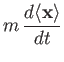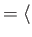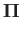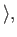(3.99)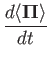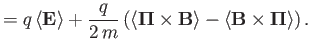(3.100)

However, the quantities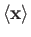,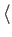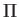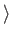,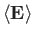,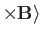, and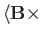are all invariant under the gauge transformation,, and. This follows because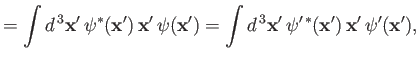(3.101)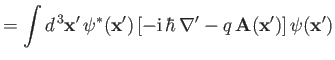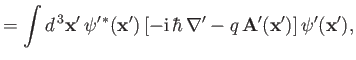(3.102)

et cetera. Thus, Equations (3.88) and (3.89) do indeed give consistent results under gauge transformation.Next: Flux Quantization and the Up: Quantum Dynamics Previous: Charged Particle Motion in
Richard Fitzpatrick 2016-01-22